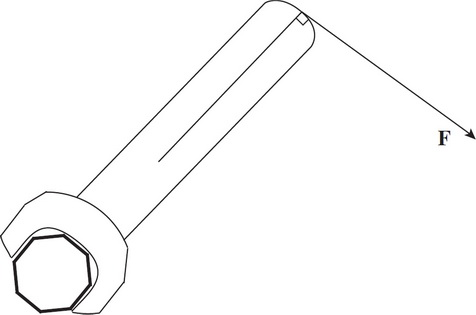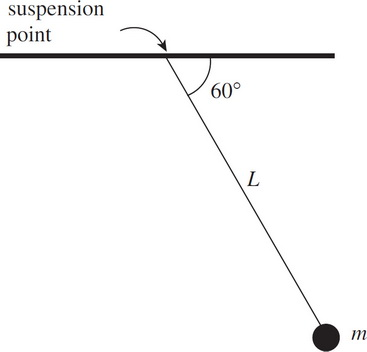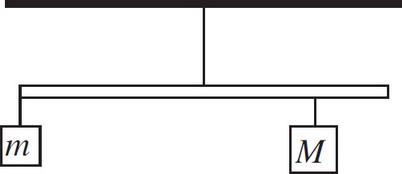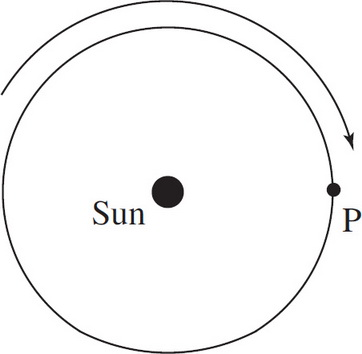# AP Physics 1: Uniform Circular Motion, Newton's Law of Gravitation, and Rotational Motion Multiple-Choice Practice Questions

### Test Information15 questions27 minutes

1. A 60 cm rope is tied to the handle of a bucket which is then whirled in a vertical circle. The mass of the bucket is 3 kg.

At the lowest point in its path, the tension in the rope is 50 N. What is the speed of the bucket?

2. A 60 cm rope is tied to the handle of a bucket which is then whirled in a vertical circle. The mass of the bucket is 3 kg.

What is the critical speed below which the rope would become slack when the bucket reaches the highest point in the circle?

3. An object moves at a constant speed in a circular path of radius r at a rate of 1 revolution per second. What is its acceleration?4. In an effort to tighten a bolt, a force F is applied as shown in the figure above. If the distance from the end of the wrench to the center of the bolt is 20 cm and F = 20 N, what is the magnitude of the torque produced by F ?5. In the figure above, what is the torque about the pendulum's suspension point produced by the weight of the bob, given that the length of the pendulum, L, is 80 cm and m = 0.50 kg ?6. A uniform meter stick of mass 1 kg is hanging from a thread attached at the stick's midpoint. One block of mass m = 3 kg hangs from the left end of the stick, and another block, of unknown mass M, hangs below the 80 cm mark on the meter stick. If the stick remains at rest in the horizontal position shown above, what is M ?

7. If the distance between two point particles is doubled, then the gravitational force between them

8. At the surface of Earth, an object of mass m has weight w. If this object is transported to an altitude that is twice the radius of Earth, then at the new location,

9. A moon of mass m orbits a planet of mass 100m. Let the strength of the gravitational force exerted by the planet on the moon be denoted by F1, and let the strength of the gravitational force exerted by the moon on the planet be F2. Which of the following is true?

10. The dwarf planet Pluto has 1/500 the mass and 1/15 the radius of Earth. What is the value of g (in m/s2) on the surface of Pluto?

11. A satellite is currently orbiting Earth in a circular orbit of radius R; its kinetic energy is K1. If the satellite is moved and enters a new circular orbit of radius 2R, what will be its kinetic energy?

12. A moon of Jupiter has a nearly circular orbit of radius R and an orbit period of T. Which of the following expressions gives the mass of Jupiter?

13. Two large bodies, Body A of mass m and Body B of mass 4m, are separated by a distance R. At what distance from Body A, along the line joining the bodies, would the gravitational force on an object be equal to zero? (Ignore the presence of any other bodies.)

14. You are looking at a top view of a planet orbiting the Sun in a clockwise direction. Which of the following would describe the velocity, acceleration, and force acting on the planet due to the Sun's pull at point P ?15. An object of mass m is traveling at constant speed v in a circular path of radius r. How much work is done by the centripetal force during one-half of a revolution?